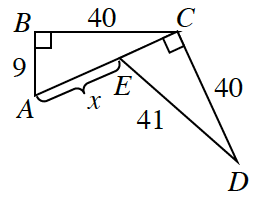### Home > INT2 > Chapter 7 > Lesson 7.2.3 > Problem7-115

7-115.

For the figure at right, determine if the two triangles are congruent. If so, create a proof (flowchart or two-column) to explain why. Then, solve for $x$. If the triangles are not congruent, explain why not.Draw the triangles separately. What is $CE$? What is $CA$?

Congruent by $HL≅$